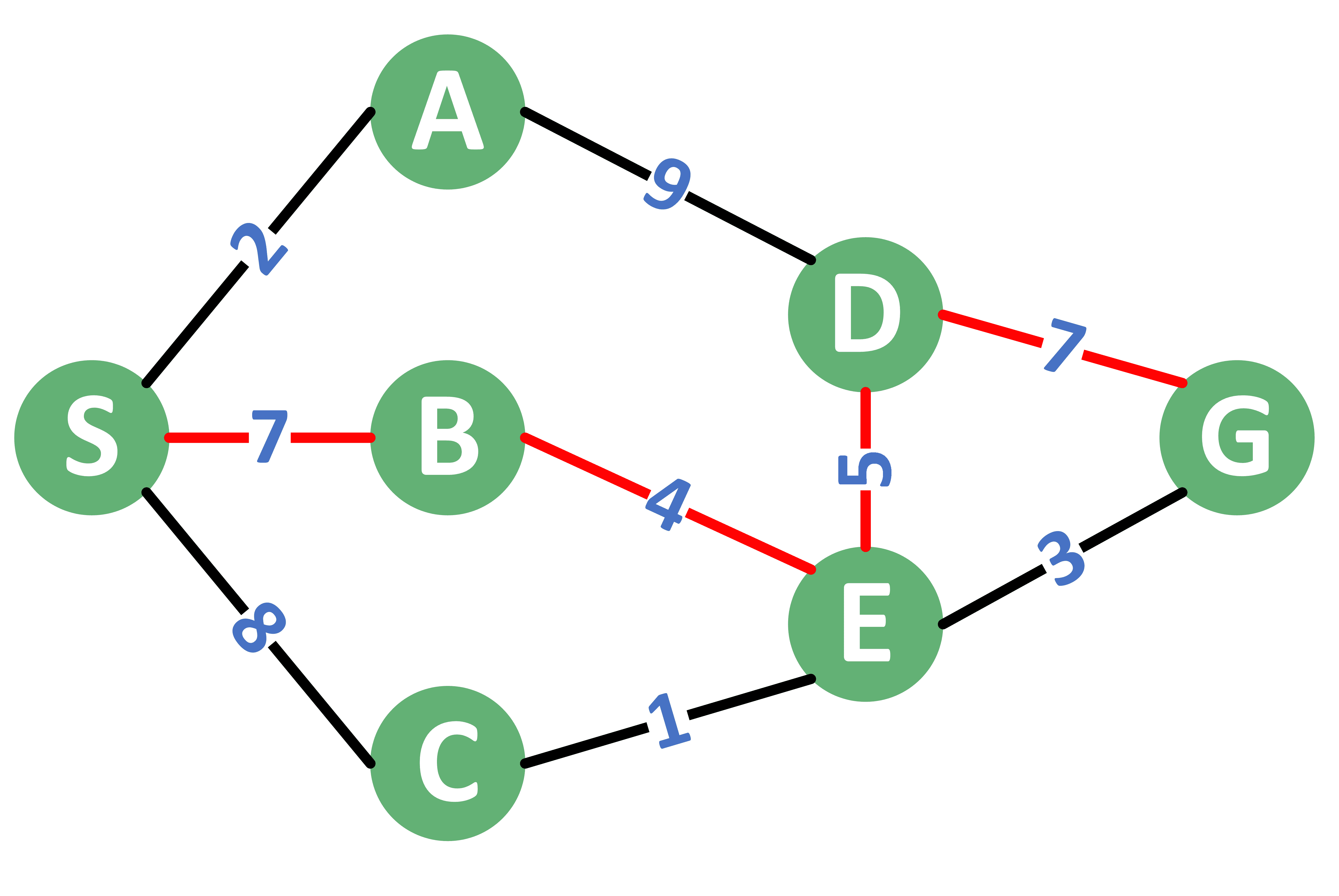## 1. Overview

In this tutorial, we’ll explain the maximum-minimum path capacity problem in graph theory. We’ll define what it is, how to solve it, and how the solution differs from Dijkstra’s.

## 2. Defining the Problem

The maximum-minimum path capacity problem deals with weighted graphs.

We consider the weight of each edge to represent that edge’s capacity. Our task is to find the path that starts from a source nodeand ends at a goal nodeinside the graph. The edge with the lowest capacity in a path forms that path’s capacity.

Among all the paths fromto, what we want to do is find the path of maximum capacity.

As a practical example, suppose the graph represents the capacity of water that each pipe can deliver per second. Obviously, we’d be looking for a path that can deliver the largest amount of water per second, and we can’t deliver it any faster than the smallest pipe in the path.

## 3. Example

Have a look at the following example. We consider the source node to beand the goal node to be.From the graph example, we see that there are multiple paths connecting nodewith. The following table lists the different paths along with their capacity.Out of all the paths, path number 3 has the largest capacity, which is 4. Therefore, the maximum-minimum capacity for the given graph equals 4.

## 4. Algorithm

In order to solve this problem, we’ll use a modified version of Dijkstra’s algorithm.

### 4.1. Differences From Dijkstra’s Algorithm

The pseudocode of this problem is mostly derived from Dijkstra’s algorithm with the following changes:

• Instead of having a distance array, we’ll have an array called. This array will store the maximum capacity of each. By the maximum capacity of a node, we mean the maximum-minimum capacity for all the paths fromto this node.
• In Dijkstra’s algorithm, we initialize each node’s distance with.
• For the slot for, we still do this, indicating that the source node has an unlimited capacity to itself.
• However, for the remaining slots, we’ll initialize thearray with, corresponding to the worst case.
• In this problem, when visiting the neighbors of some node, the concept of updating the capacity of a neighboring node is different from the one in Dijkstra’s algorithm. In our case, we update the capacity of each neighboring node with the maximum value among the previous value, the capacity of the node that got us to this neighboring node, and the capacity of the edge we just crossed. Also, we’ll need to update this neighboring node’s capacity inside the data structure we use.
• In each step, rather than choosing the node with the lowest cost, we’ll choose the node with the largest capacity.

The proof of this algorithm’s optimality is that we always process the node with the largest capacity. Hence, when we reach the goal node, all the remaining nodes inside the data structure must have a smaller capacity. Therefore, the found path would be the one with the maximum-minimum capacity.

### 4.2. Pseudocode

Now, let’s review the algorithm in pseudocode:First, we initialize thearray with. We’ll also initialize an arraywhich will store, for each node, the node that gets us to reach nodewith the maximum path capacity.

Next, we initialize the capacity of the source node withand add all the nodes to. The purpose ofis to find the node with the maximum capacity so far quickly. Also,needs to support updating the capacity of some node through the function. For this, we can use a set that stores the node with its capacity sorted in descending order based on the capacity.

In each step, we extract the node with the maximum path capacity fromusing the function. If the extracted node has a capacity equal to, then all the remaining nodes insidecan’t be reached fromnode. Therefore, we can just stop processing further nodes.

Otherwise, we iterate over all the neighbors of node. for each neighbor, we calculate the new capacity. The new capacity is the minimum between the capacity of nodeand the capacity of the edge betweenand. Therefore, we’ve taken the minimum capacity along the path.

After that, we compare the new capacity to the old one for node. If the new capacity is better, then we update the information of nodewith the new capacity. Updating the information of nodeincludes updating its capacity, updating thevalue for it to become, and using the functionthat removes this node’s object fromand adds it again with the new path capacity.

Finally, we return, which is the calculated capacity.

The algorithm can be implemented to have a complexity of, similar to Dijkstra’s algorithm, whereis the number of edges inside the graph andis the number of vertices.

### 4.3. Finding the Path

We’ll use thearray to restore the path we need. Let’s take a look at the algorithm:freestar.config.enabled_slots.push({ placementName: "baeldung_incontent_1", slotId: "baeldung_incontent_1" });



The algorithm is simple. We start from thenode. In each step, we add the current node to the path. Also, we move one step back to the previous node using thearray calculated infunction.

Since thenode will have its previous node equal to, we can stop going back once we reach thevalue.

The complexity of this algorithm (without the findMaxMin function) is, whereis the total number of vertices inside the graph.

## 5. Conclusion

In this tutorial, we presented the maximum-minimum capacity in a graph problem. First, we defined the problem. Next, we provided an example that better explains the concept.

After that, we highlighted the main differences between our algorithm and Dijkstra’s algorithm.

Finally, we showed the algorithm.